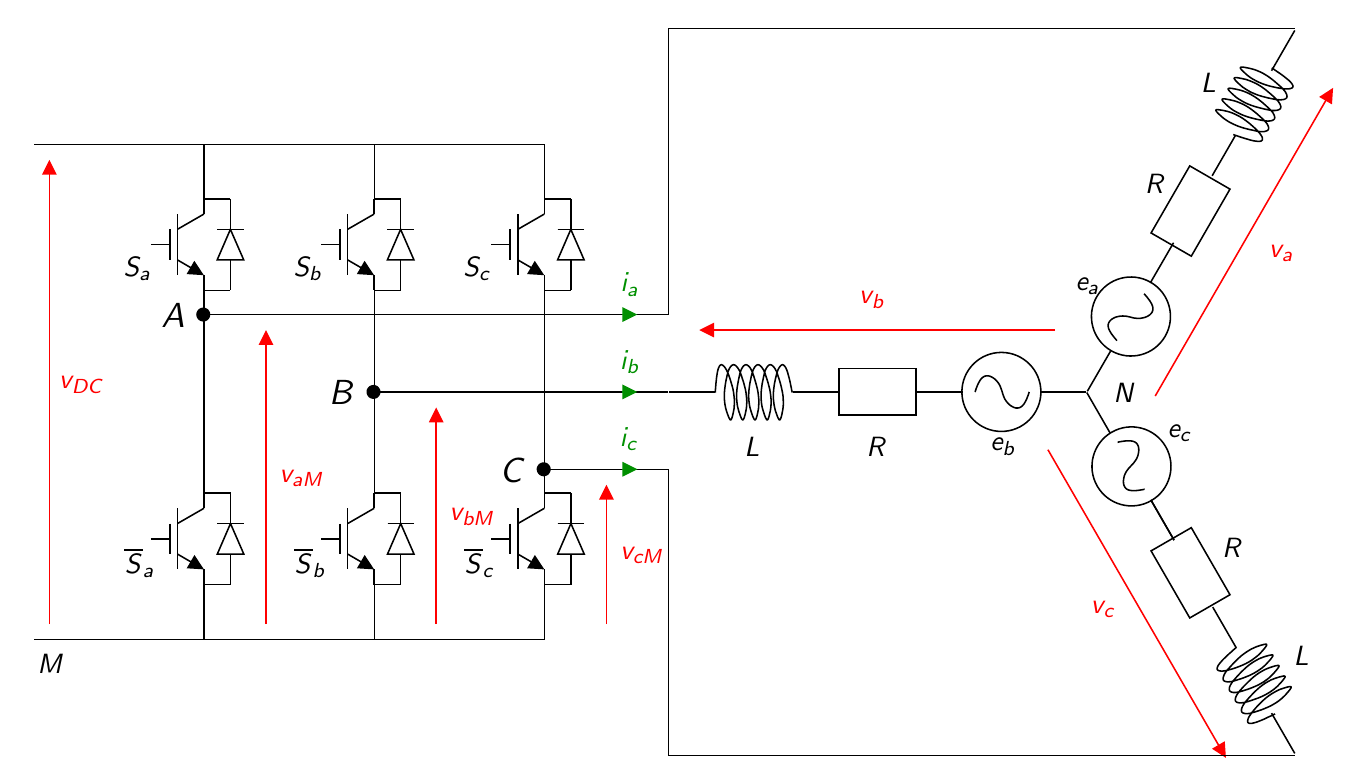# Motor Control University

## PMSM modeling

The motor considered here is a three-phase, star-connected motor. The motor is assumed to be electrically balanced. Magnetic saturations are not taken into account (inductances are independent of current). Finally, the EMF (Electro-Mechanical Forces) are sinusoidal.

### Operative part scheme

The figure below represents the whole system including the motor and its inverter.The system can be described with the following bloc diagram.

### Classical $$abc$$ frame

The electrical equation in the $$abc$$ frame is given by: $L\frac{di_{abc}}{dt} = v_{abc}-R i_{abc}- e_{abc},$ with: $e_{abc}= -p\phi_f\omega \begin{bmatrix}\sin(p\theta)\\\sin(p\theta-\frac{2\pi}{3})\\\sin(p\theta+\frac{2\pi}{3})\end{bmatrix},$ where $$i_{abc} = \left[\begin{matrix}i_a & i_b & i_c\end{matrix}\right]^\intercal$$ is the stator phase current, $$v_{abc} = \left[\begin{matrix}v_a & v_b & v_c\end{matrix}\right]^\intercal$$ the stator phase voltage, $$\omega$$ and $$\theta$$ are the speed and position respectively and $$p$$ the pole pair number. $$L$$ and $$R$$ and the stator inductance and resistor, $$\phi_f$$ the flux constant.

The mechanical equations are given by: $\begin{array}{lcl} J \frac{d\omega}{dt}&=&\tau_m-f_v\omega -\tau_l,\\ \frac{d\theta}{dt} &=& \omega, \end{array}$ where J is the inertia, $$f_v$$ is the viscous friction coefficient. The electromechanical torque is denoted $$\tau_m$$ given by : $\tau_m = \frac{1}{\omega} e_{abc}^\intercal i_{abc}.$ and $$\tau_l$$ the load torque.